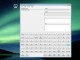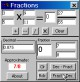Scroll Decimal 1.0 Scroll Decimal is a FaceSpan application which shows the decimal equivalent for fractions. FreewareIP to Decimal 1.0 IP to Decimal is a small utility for converting IP Addresses (i. FreewareHex&Decimal 1.0 Hex&Decimal is a very simple script that does 1 of 2 things. FreewareDecimal Ease 1.0.1 Decimal Ease is a program for converting decimal yards, feet, inches to yards, feet, inches and fractions or vice versa, if you are an Architect, Draftsman, Engineer, Designer or need to work with decimal yards, feet or inches then you will find this program of immense use. SharewareDecimal Time Project Clock 1.0 The DTP Dashboard widget calculates the date and time in a decimal format and displays it. FreewareScientific Calculator Decimal 1.0.1.5 Scientific Calculator Decimal for scientists, engineers, teachers, and students. SharewareDeciTime 1.0 DeciTime is a fun application sports a new base for measuring the day. FreewareFractions n Decimals CE 5.3 Add, Subtract, Divide and Multiply fractions. Convert decimals/fractions CE 2.11 SharewareDecimal 1.2 Swing Java application (multi-platform) that translates an IP address (127. GPLDeciCalc 1.0 DeciCalc is a quick calculator for figuring out the decimal equivalents of common fractions. Freeware